# Thread: Table of Integrals/Trigonometic Substitution and Partial Fraction Decomposition

1. ## Table of Integrals/Trigonometic Substitution and Partial Fraction Decomposition

Hey guys, I have a few problems that I'm having trouble with. If anyone could help, I would really appreciate it.

Here are the instructions:

Problem 1. Each of these problems requires an integral that can be calculated either by making a trigonometric substitution or by using a Table of Integrals. In each case, do the following:

i) Write the integral, including the limits of integration, that will produce the required quantity.

ii) Write an antiderivative for your integral. You may do this by direct calculation, or you may use a Table of Integrals.

iii) Complete the calculation to compute the required quantity.

a) Calculate the length along the curve y = ln(x), 1 <= x <= e

My Solution

ai) $\displaystyle y = ln(x), \frac{dy}{dx} = \frac{1}{x}$

$\displaystyle \int^e_1\sqrt{1 + \frac{1}{x^2}}$ dx

aii) Here, I don't know what to do. I can't find any form in my textbook's table of integrals that matches this, nor do I know where to start by using a trigonometric substitution. Help please!

b) Calculate the length along the parabola $\displaystyle y = x^2$ between the points (0,0) and (1,1).

My Solution

bi) $\displaystyle y = x^2, \frac{dy}{dx} = 2x$

$\displaystyle \int^1_0\sqrt{1 + 4x^2}$ dx

bii) Once again, I can't figure out what to do here. I tried substituting $\displaystyle \tan\theta$ for 2x, but it isn't working out right. Help please!

c) Calculate the area bounded by the ellipse $\displaystyle \frac{x^2}{a^2} + \frac{y^2}{b^2} = 1$.

My Solution

ci) $\displaystyle \frac{x^2}{a^2} + \frac{y^2}{b^2} = 1, y = \sqrt{b - \frac{bx}{a}}$

$\displaystyle \int^a_{-a}\sqrt{b - \frac{bx}{a}}$ dx

cii) I'm in the same boat here, I have no idea what to do. Help please!

Problem 2. Make a complete calculation of $\displaystyle \int\frac{3x^6 + 27x^4 + 2x^3 + 2x^2 + 45}{x^4 + 9x^2}$ dx.

= $\displaystyle \int\frac{3x^6 + 27x^4 + 2x^3 + 2x^2 + 45}{x^2(x^2 + 9)}$ dx = $\displaystyle \int(\frac{A}{x} + \frac{B}{x^2} + \frac{Cx + D}{x^2 + 9})$

$\displaystyle 3x^6 + 27x^4 + 2x^3 + 2x^2 + 45 = A(x)(x^2 + 9) + B(x^2 + 9) + (Cx + D)(x^2)$ = $\displaystyle (A + C)x^3 + (B + D)x^2 + (9A)x + 9B$

A + C = 2, B + D = 2, 9A = 0, 9B = 45

A = 0, B = 5, C = 2, D = -3

= $\displaystyle \int(\frac{5}{x^2} + \frac{2x - 3}{x^2 + 9})$ dx = $\displaystyle \int\frac{5}{x^2}$ dx + $\displaystyle \int\frac{2x}{x^2 + 9}$ dx - $\displaystyle \int\frac{3}{x^2 + 9}$ dx = $\displaystyle 5ln(x^2) + ln(x^2 + 9) - 3ln(x^2 + 9) + C$

Could someone check to see if I did this problem correctly? Thank you very much in advance.

2. Hint for ai: (1/x^2)=(1/x)^2

3.Originally Posted by mturner07Problem 2. Make a complete calculation of $\displaystyle \int\frac{3x^6 + 27x^4 + 2x^3 + 2x^2 + 45}{x^4 + 9x^2}$ dx.

= $\displaystyle \int\frac{3x^6 + 27x^4 + 2x^3 + 2x^2 + 45}{x^2(x^2 + 9)}$ dx = $\displaystyle \int(\frac{A}{x} + \frac{B}{x^2} + \frac{Cx + D}{x^2 + 9})$

$\displaystyle 3x^6 + 27x^4 + 2x^3 + 2x^2 + 45 = A(x)(x^2 + 9) + B(x^2 + 9) + (Cx + D)(x^2)$ = $\displaystyle (A + C)x^3 + (B + D)x^2 + (9A)x + 9B$

A + C = 2, B + D = 2, 9A = 0, 9B = 45

A = 0, B = 5, C = 2, D = -3

= $\displaystyle \int(\frac{5}{x^2} + \frac{2x - 3}{x^2 + 9})$ dx = $\displaystyle \int\frac{5}{x^2}$ dx + $\displaystyle \int\frac{2x}{x^2 + 9}$ dx - $\displaystyle \int\frac{3}{x^2 + 9}$ dx = $\displaystyle 5ln(x^2) + ln(x^2 + 9) - 3ln(x^2 + 9) + C$

Could someone check to see if I did this problem correctly? Thank you very much in advance.
You can't apply partial fractions here until the fraction is proper! Apply long division or synthetic division. Then apply partial fractions to the remainder.

4.Originally Posted by mturner07a) Calculate the length along the curve y = ln(x), 1 <= x <= e

My Solution

ai) $\displaystyle y = ln(x), \frac{dy}{dx} = \frac{1}{x}$

$\displaystyle \int^e_1\sqrt{1 + \frac{1}{x^2}}$ dx
Just in case a picture helps...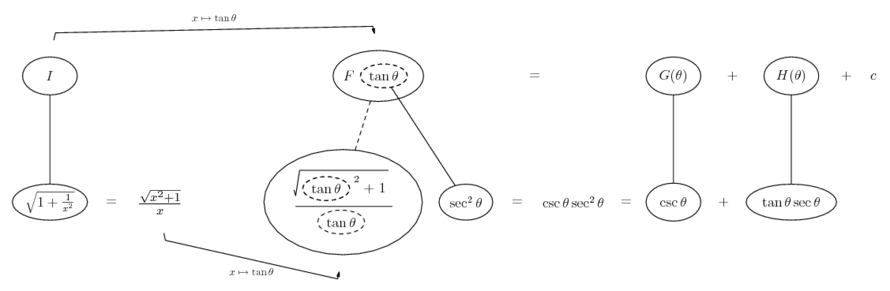... where... is the chain rule. Straight continuous lines differentiate downwards (integrate up) with respect to x, and the straight dashed line similarly but with respect to the dashed balloon expression (the inner function of the composite which is subject to the chain rule).

The general drift is...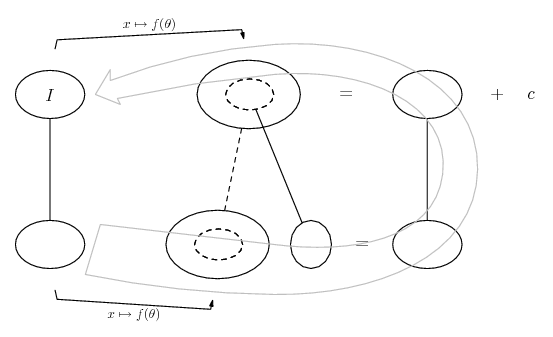And the rest...
Spoiler: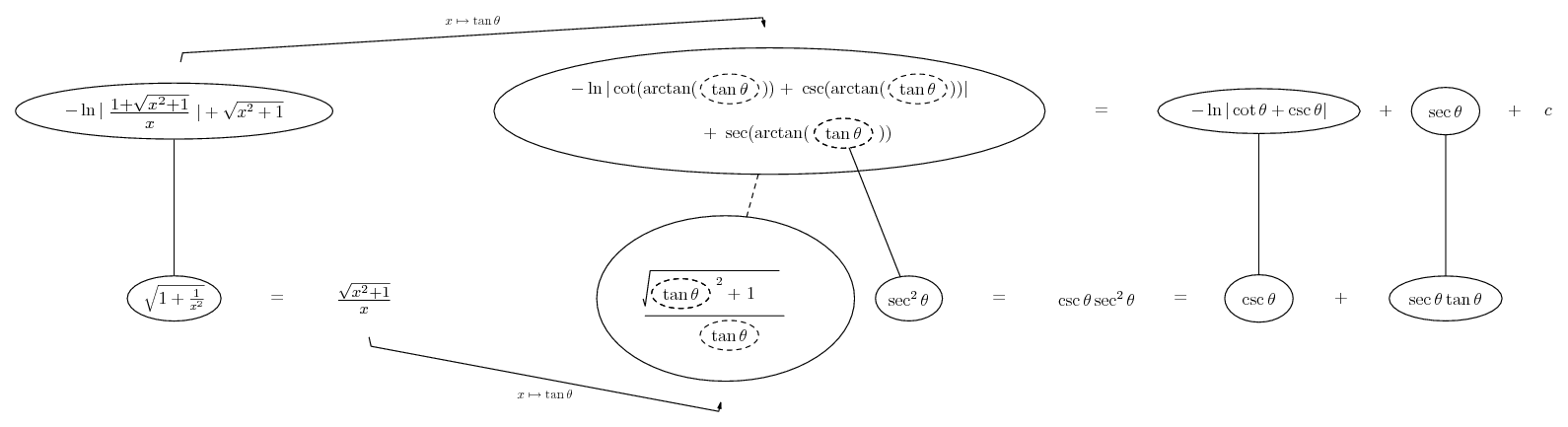Originally Posted by mturner07b) Calculate the length along the parabola $\displaystyle y = x^2$ between the points (0,0) and (1,1).

My Solution

bi) $\displaystyle y = x^2, \frac{dy}{dx} = 2x$

$\displaystyle \int^1_0\sqrt{1 + 4x^2}$ dx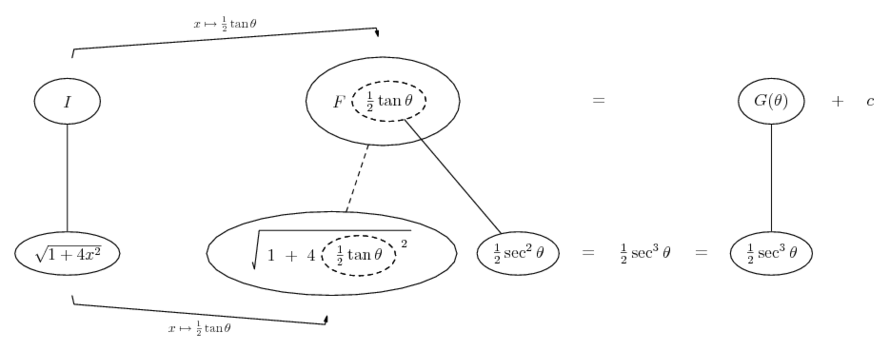Spoiler: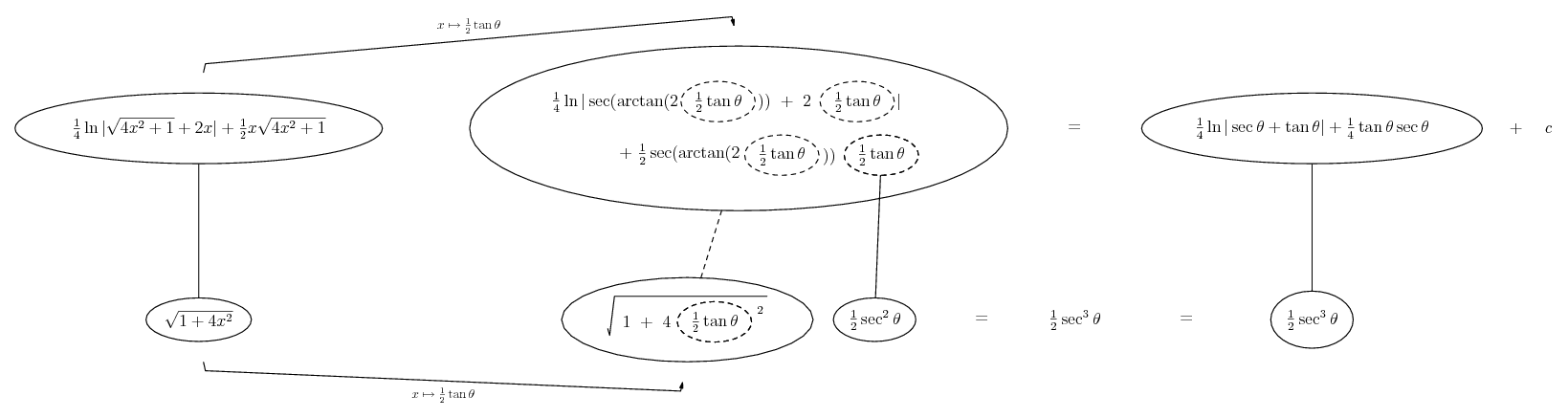Originally Posted by mturner07c) Calculate the area bounded by the ellipse $\displaystyle \frac{x^2}{a^2} + \frac{y^2}{b^2} = 1$.

My Solution

ci) $\displaystyle \frac{x^2}{a^2} + \frac{y^2}{b^2} = 1, y = \sqrt{b - \frac{bx}{a}}$

$\displaystyle \int^a_{-a}\sqrt{b - \frac{bx}{a}}$ dx
Use $\displaystyle 2b \int^a_{-a}\sqrt{1 - \frac{x^2}{a^2}}$ dx (because only each half of the ellipse is a function).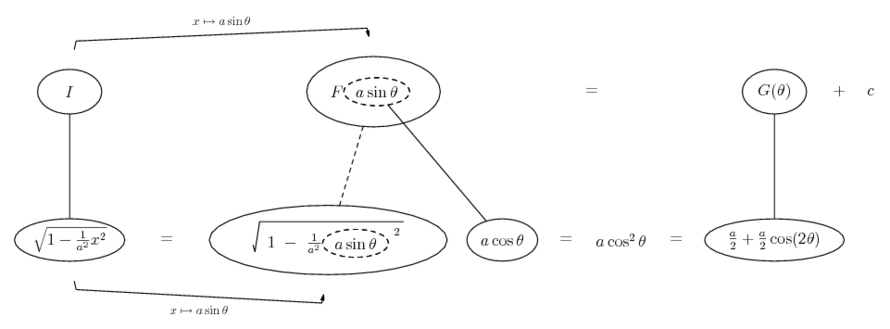Spoiler: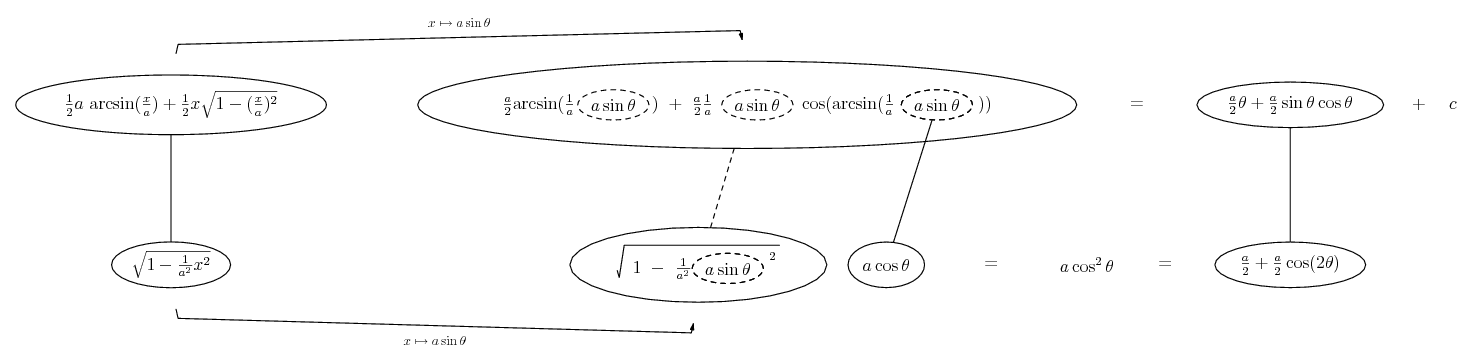_________________________________________
Don't integrate - balloontegrate!

Balloon Calculus; standard integrals, derivatives and methods

Balloon Calculus Drawing with LaTeX and Asymptote!

#### Search Tags

decomposition, fraction, integrals or trigonometic, partial, substitution, table